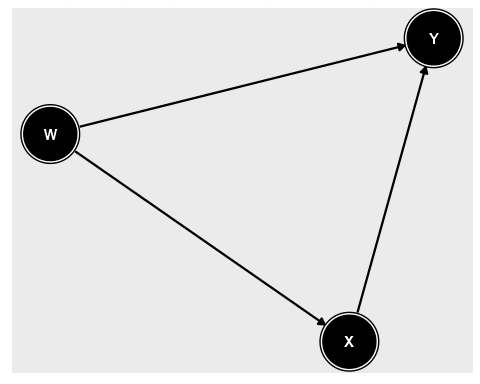# Lecture 18 Closing Back Doors: Controlling

## Recap

• We discussed how to draw a causal diagram
• How to identify the front and back door paths
• And how we can close those back door paths by controlling/adjusting in order to identify the front-door paths we want!
• And so we get our causal effect

## Today

• Today we’re going to be going a little deeper into what it means to actually control/adjust for things
• And we’re also going to talk about times when controlling/adjusting makes things WORSE - collider bias!
• I’m going to just start saying “controlling”, by the way - “adjusting” is a little more accurate, but “controlling” is more common

## Controlling

• Up to now, here’s how we’ve been getting the relationship between `X` and `Y` while controlling for `W`:
1. See what part of `X` is explained by `W`, and subtract it out. Call the result the residual part of `X`.
2. See what part of `Y` is explained by `W`, and subtract it out. Call the result the residual part of `Y`.
3. Get the relationship between the residual part of `X` and the residual part of `Y`.
• With the last step including things like getting the correlation, plotting the relationship, calculating the variance explained, or comparing mean `Y` across values of `X`

## In code

``````df <- tibble(w = rnorm(100)) %>%
mutate(x = 2*w + rnorm(100)) %>%
mutate(y = 1*x + 4*w + rnorm(100))
cor(df\$x,df\$y)``````
``##  0.9479742``
``````df <- df %>% group_by(cut(w,breaks=5)) %>%
mutate(x.resid = x - mean(x),
y.resid = y - mean(y))
cor(df\$x.resid,df\$y.resid)``````
``##  0.7367752``

## In Diagrams

• The relationship between `X` and `Y` reflects both `X->Y` and `X<-W->Y`
• We remove the part of `X` and `Y` that `W` explains to get rid of `X<-W` and `W->Y`, blocking `X<-W->Y` and leaving `X->Y`## More than One Variable

• It’s quite possible to control for more than one variable at a time
• Although we won’t be doing it much in this class
• A common way to do this is called multiple regression
• You can do it with our method too, but it gets tedious pretty quickly

## More than One Variable

``````df <- tibble(w = rnorm(100),v=rnorm(100)) %>%
mutate(x = 2*w + 3*v + rnorm(100)) %>%
mutate(y = 1*x + 4*w + 1.5*v + rnorm(100))
cor(df\$x,df\$y)``````
``##  0.9340934``
``````df <- df %>% group_by(cut(w,breaks=5)) %>%
mutate(x.resid = x - mean(x),
y.resid = y - mean(y)) %>%
group_by(cut(v,breaks=5)) %>%
mutate(x.resid2 = x.resid - mean(x.resid),
y.resid2 = y.resid - mean(y.resid))
cor(df\$x.resid2,df\$y.resid2)``````
``##  0.7419072``